# Softmax Classifiers Explained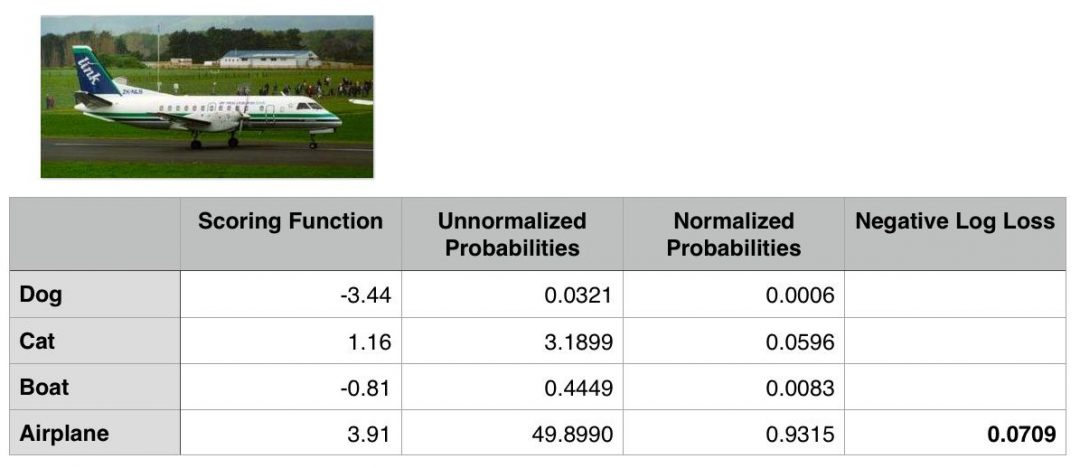Last week, we discussed Multi-class SVM loss; specifically, the hinge loss and squared hinge loss functions.

A loss function, in the context of Machine Learning and Deep Learning, allows us to quantify how “good” or “bad” a given classification function (also called a “scoring function”) is at correctly classifying data points in our dataset.

However, while hinge loss and squared hinge loss are commonly used when training Machine Learning/Deep Learning classifiers, there is another method more heavily used…

In fact, if you have done previous work in Deep Learning, you have likely heard of this function before — do the terms Softmax classifier and cross-entropy loss sound familiar?

I’ll go as far to say that if you do any work in Deep Learning (especially Convolutional Neural Networks) that you’ll run into the term “Softmax”: it’s the final layer at the end of the network that yields your actual probability scores for each class label.

Looking for the source code to this post?

## Softmax Classifiers Explained

While hinge loss is quite popular, you’re more likely to run into cross-entropy loss and Softmax classifiers in the context of Deep Learning and Convolutional Neural Networks.

Why is this?

Simply put:

Softmax classifiers give you probabilities for each class label while hinge loss gives you the margin.

It’s much easier for us as humans to interpret probabilities rather than margin scores (such as in hinge loss and squared hinge loss).

Furthermore, for datasets such as ImageNet, we often look at the rank-5 accuracy of Convolutional Neural Networks (where we check to see if the ground-truth label is in the top-5 predicted labels returned by a network for a given input image).

Seeing (1) if the true class label exists in the top-5 predictions and (2) the probability associated with the predicted label is a nice property.

### Understanding Multinomial Logistic Regression and Softmax Classifiers

The Softmax classifier is a generalization of the binary form of Logistic Regression. Just like in hinge loss or squared hinge loss, our mapping function f is defined such that it takes an input set of data x and maps them to the output class labels via a simple (linear) dot product of the data x and weight matrix W:$f(x_{i}, W) = Wx_{i}$

However, unlike hinge loss, we interpret these scores as unnormalized log probabilities for each class label — this amounts to swapping out our hinge loss function with cross-entropy loss: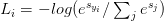$L_{i} = -log(e^{s_{y_{i}}} / \sum_{j} e^{s_{j}})$

So, how did I arrive here? Let’s break the function apart and take a look.

To start, our loss function should minimize the negative log likelihood of the correct class: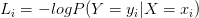$L_{i} = -log P(Y=y_{i}|X=x_{i})$

This probability statement can be interpreted as: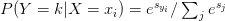$P(Y=k|X=x_{i}) = e^{s_{y_{i}}} / \sum_{j} e^{s_{j}}$

Where we use our standard scoring function form: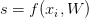$s = f(x_{i}, W)$

As a whole, this yields our final loss function for a single data point, just like above:$L_{i} = -log(e^{s_{y_{i}}} / \sum_{j} e^{s_{j}})$

Note: Your logarithm here is actually base e (natural logarithm) since we are taking the inverse of the exponentiation over e earlier.

The actual exponentiation and normalization via the sum of exponents is our actual Softmax function. The negative log yields our actual cross-entropy loss.

Just as in hinge loss or squared hinge loss, computing the cross-entropy loss over an entire dataset is done by taking the average: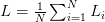$L = \frac{1}{N} \sum^{N}_{i=1} L_{i}$

If these equations seem scary, don’t worry — I’ll be working an actual numerical example in the next section.

Note: I’m purposely leaving out the regularization term as to not bloat this tutorial or confuse readers. We’ll return to regularization and explain what it is, how to use, and why it’s important for machine learning/deep learning in a future blog post.

### A worked Softmax example

To demonstrate cross-entropy loss in action, consider the following figure: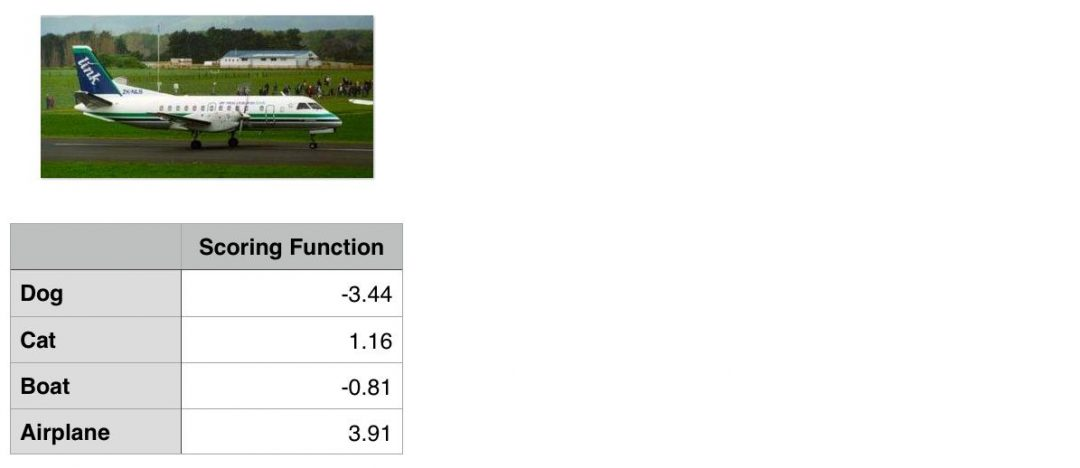Figure 1: To compute our cross-entropy loss, let’s start with the output of our scoring function (the first column).

Our goal is to classify whether the image above contains a dogcatboat, or airplane.

Clearly we can see that this image is an “airplane”. But does our Softmax classifier?

To find out, I’ve included the output of our scoring function f for each of the four classes, respectively, in Figure 1 above. These values are our unnormalized log probabilities for the four classes.

Note: I used a random number generator to obtain these values for this particular example. These values are simply used to demonstrate how the calculations of the Softmax classifier/cross-entropy loss function are performed. In reality, these values would not be randomly generated — they would instead be the output of your scoring function f.

Let’s exponentiate the output of the scoring function, yielding our unnormalized probabilities: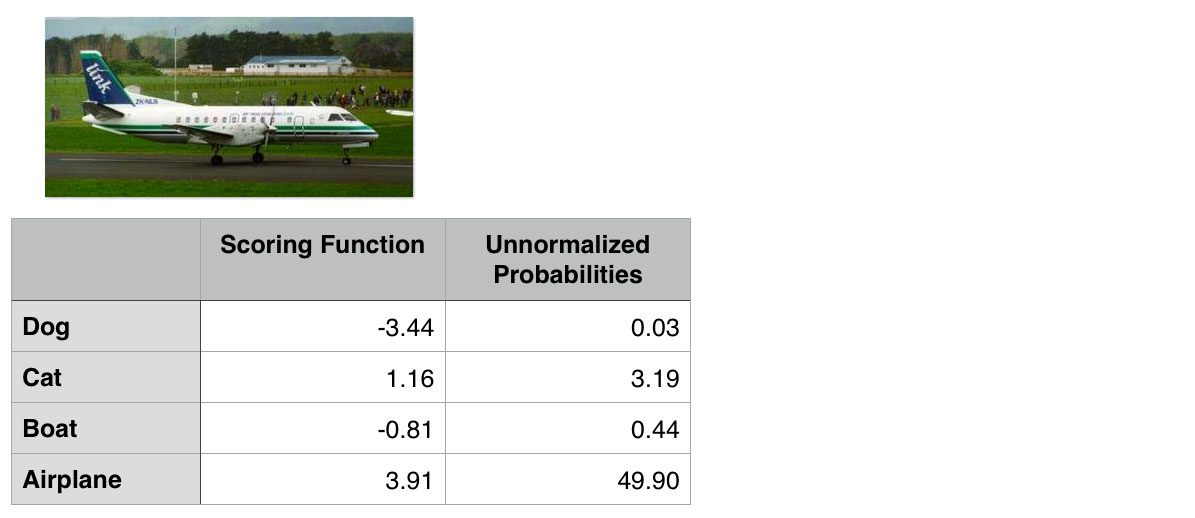Figure 2: Exponentiating the output values from the scoring function gives us our unnormalized probabilities.

The next step is to take the denominator, sum the exponents, and divide by the sum, thereby yielding the actual probabilities associated with each class label: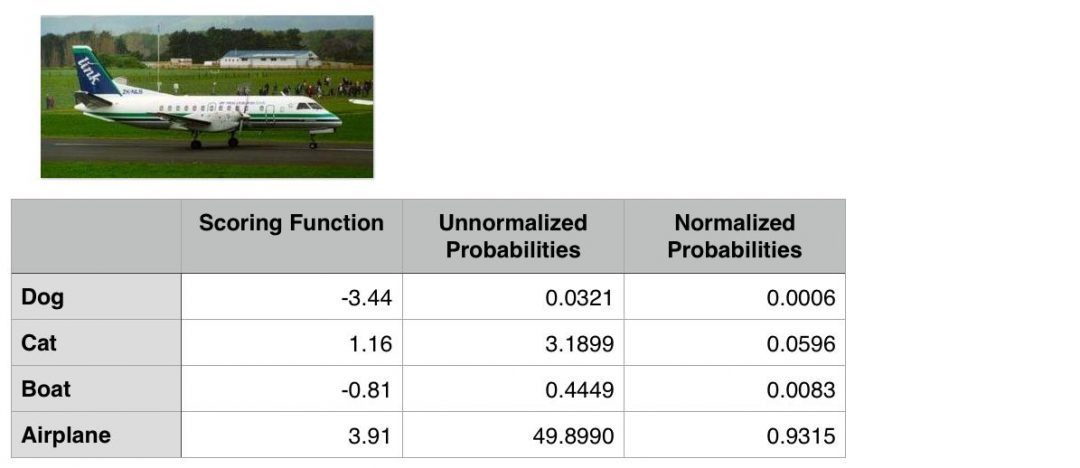Figure 3: To obtain the actual probabilities, we divide each individual unnormalized probability by the sum of unnormalized probabilities.

Finally, we can take the negative log, yielding our final loss:Figure 4: Taking the negative log of the probability for the correct ground-truth class yields the final loss for the data point.

In this case, our Softmax classifier would correctly report the image as airplane with 93.15% confidence.

### The Softmax Classifier in Python

In order to demonstrate some of the concepts we have learned thus far with actual Python code, we are going to use a SGDClassifier with a log loss function.

For this example, we’ll once again be using the Kaggle Dogs vs. Cats dataset, so before we get started, make sure you have:

In our particular example, the Softmax classifier will actually reduce to a special case — when there are K=2 classes, the Softmax classifier reduces to simple Logistic Regression. If we have > 2 classes, then our classification problem would become Multinomial Logistic Regression, or more simply, a Softmax classifier.

With that said, open up a new file, name it softmax.py , and insert the following code:

If you’ve been following along on the PyImageSearch blog over the past few weeks, then the code above likely looks fairly familiar — all we are doing here is importing our required Python packages.

We’ll be using the scikit-learn library, so if you don’t already have it installed, be sure to install it now:

We’ll also be using my imutils package, a series of convenience functions used to make performing common image processing operations an easier task. If you do not have imutils  installed, you’ll want to install it as well:

Next, we define our extract_color_histogram  function which is used to quantify the color distribution of our input image  using the supplied number of bins :

I’ve already reviewed this function a few times before, so I’m going to skip the detailed review. For a more thorough discussion of extract_color_histogram , why we are using it, and how it works, please see this blog post.

In the meantime, simply keep in mind that this function quantifies the contents of an image by constructing a histogram over the pixel intensities.

Let’s parse our command line arguments and grab the paths to our 25,000 Dogs vs. Cats images from disk:

We only need a single switch here, --dataset , which is the path to our input Dogs vs. Cats images.

Once we have the paths to these images, we can loop over them individually and extract a color histogram for each image:

Again, since I have already reviewed this boilerplate code multiple times on the PyImageSearch blog, I’ll refer you to this blog post for a more detailed discussion on the feature extraction process.

Our next step is to construct the training and testing split. We’ll use 75% of the data for training our classifier and the remaining 25% for testing and evaluating the model:

We also train our SGDClassifier  using the log  loss function (Lines 75 and 76). Using the log  loss function ensures that we’ll obtain probability estimates for each class label at testing time.

Lines 79-82 then display a nicely formatted accuracy report for our classifier.

To examine some actual probabilities, let’s loop over a few randomly sampled training examples and examine the output probabilities returned by the classifier:

Note: I’m randomly sampling from the training data rather than the testing data to demonstrate that there should be a noticeably large gap in between the probabilities for each class label. Whether or not each classification is correct is a a different story — but even if our prediction is wrong, we should still see some sort of gap that indicates that our classifier is actually learning from the data.

Line 93 handles computing the probabilities associated with the randomly sampled data point via the .predict_proba  function.

The predicted probabilities for the cat and dog class are then displayed to our screen on Lines 97 and 98.

### Softmax Classifier Results

Once you have:

You can execute the following command to extract features from our dataset and train our classifier:

After training our SGDClassifier , you should see the following classification report: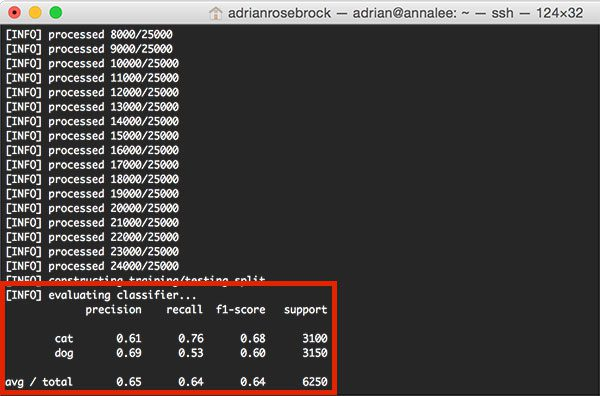Figure 5: Computing the accuracy of our SGDClassifier with log loss — we obtain 65% classification accuracy.

Notice that our classifier has obtained 65% accuracy, an increase from the 64% accuracy when utilizing a Linear SVM in our linear classification post.

To investigate the individual class probabilities for a given data point, take a look at the rest of the softmax.py  output: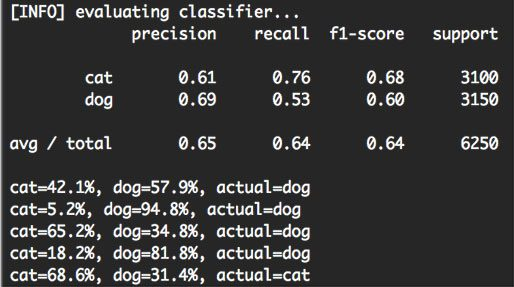Figure 6: Investigating the class label probabilities for each prediction.

For each of the randomly sampled data points, we are given the class label probability for both “dog” and “cat”, along with the actual ground-truth label.

Based on this sample, we can see that we obtained 4 / 5 = 80% accuracy.

But more importantly, notice how there is a particularly large gap in between class label probabilities. If our Softmax classifier predicts “dog”, then the probability associated with “dog” will be high. And conversely, the class label probability associated with “cat” will be low.

Similarly, if our Softmax classifier predicts “cat”, then the probability associated with “cat” will be high, while the probability for “dog” will be “low”.

This behavior implies that there some actual confidence in our predictions and that our algorithm is actually learning from the dataset.

Exactly how the learning takes place involves updating our weight matrix W, which boils down to being an optimization problem. We’ll be reviewing how to perform gradient decent and other optimization algorithms in future blog posts.

## Summary

In today’s blog post, we looked at the Softmax classifier, which is simply a generalization of the the binary Logistic Regression classifier.

When constructing Deep Learning and Convolutional Neural Network models, you’ll undoubtedly run in to the Softmax classifier and the cross-entropy loss function.

While both hinge loss and squared hinge loss are popular choices, I can almost guarantee with absolute certainly that you’ll see cross-entropy loss with more frequency — this is mainly due to the fact that the Softmax classifier outputs probabilities rather than margins. Probabilities are much easier for us as humans to interpret, so that is a particularly nice quality of Softmax classifiers.

Now that we understand the fundamentals of loss functions, we’re ready to tack on another term to our loss method — regularization.

The regularization term is appended to our loss function and is used to control how our weight matrix W “looks”. By controlling W and ensuring that it “looks” a certain way, we can actually increase classification accuracy.

After we discuss regularization, we can then move on to optimization — the process that actually takes the output of our scoring and loss functions and uses this output to tune our weight matrix W to actually “learn”.

Anyway, I hope you enjoyed this blog post!If you would like to download the code and images used in this post, please enter your email address in the form below. Not only will you get a .zip of the code, I’ll also send you a FREE 17-page Resource Guide on Computer Vision, OpenCV, and Deep Learning. Inside you'll find my hand-picked tutorials, books, courses, and libraries to help you master CV and DL! Sound good? If so, enter your email address and I’ll send you the code immediately!

### 12 Responses to Softmax Classifiers Explained

1.Sujit Pal September 12, 2016 at 12:24 pm #

Nice tutorial, very nicely explained. Shouldn’t the negative log loss for the airplane output be log base e (since it is the inverse of exponentiation over e earlier)? The value shown seems to be log base 10.

•Adrian Rosebrock September 12, 2016 at 12:43 pm #

I used an Excel spreadsheet to derive this example. The negative log loss for the airplane was computed by:

-log("Normalized Probabilities")

Which does indeed yield 0.0308. The “Normalized Probabilities” are also derived by taking the “Unnormalized Probabilities” and dividing by the sum of the column. Unless I’m having a brain fart (totally possible), this seems correct.

EDIT: You’re absolutely right. My confusion came from not understanding which log function was being used by default inside the spreadsheet. I have updated the post and example image to reflect this change. Thanks for catching the error!

2.Flavia September 13, 2016 at 1:09 pm #

I get the following error:

ValueError: setting an array element with a sequence.

when i run this line of code:

model.fit(trainData, trainLabels)

Note: I’m note using the Kaggle image dataset but rather my own.

Searches on google indicate that i might be using an outdated version of sklearn… but i highly doubt this is the problem. Any assistance/pointers are greatly appreciated.

•Adrian Rosebrock September 15, 2016 at 9:40 am #

Without knowing what your dataset is, the directory structure of the dataset, or the labels, it’s pretty challenging to diagnose what the problem is.

However, if you are getting an “setting an array element with a sequence” error, the error is with either trainData or trainLabels (and potentially both).

The trainData should contain your feature vectors, one feature vector for every image in your dataset. Therefore, this array should be 2D.

The trainLabels list should be 1D — one entry for each data point in your dataset.

I would go back and confirm that you have extracted features and class labels properly.

3.Robert September 20, 2016 at 7:46 am #

Aren’t you testing the model on the training set here?

Regards,
Robert

•Adrian Rosebrock September 20, 2016 at 9:30 am #

Yes, that is correct. You normally wouldn’t do that, but as I stated in the blog post, I wanted to demonstrate that the model is actually learning which is demonstrated by the large gaps in probabilities between the two classes. This model isn’t powerful enough to demonstrate those same large gaps on the testing data (that will come in future blog posts).

4.Aditya December 14, 2016 at 9:01 pm #

While computing dE/dw for a particular weight using softmax and log-loss I got a strange result which indicates that for all weights connected to a node in the previous layer the update value is same. Something like: dw_(i,j) = -z_(i,j)*op_j + -z_(i,j)

All the weights are initialized randomly, does this not introduce a bias in the network..

5.vinay kumar October 17, 2017 at 8:45 am #

tutorial on softmax and other activation functions:
https://quickkt.com/tutorials/artificial-intelligence/deep-learning/activation-function/

6.Ram April 5, 2018 at 11:06 am #

Hi,

Thanks for clear explanation. Can u please explain where the input scoring functions are calculated and provide input to softmax function

•Adrian Rosebrock April 6, 2018 at 8:53 am #

The scoring function is arbitrary for this example. Normally they would be the output predictions of whatever your machine learning model is. For a simple NN this might be the product followed by an activation function. If you’re interested in learning more about parameterized learning, scoring functions, loss functions, etc., I would recommend taking a look at Deep Learning for Computer Vision with Python. Inside the book I have multiple chapters dedicated to this very topic.

7.ravi December 25, 2018 at 5:01 am #

Hello..
Thanks for such a nice tutorial. I run the code as a tease but it throws an error given below

(catProb, dogProb) = model.predict_proba(hist)
ValueError: too many values to unpack

if i run the code without “.predict_proba”. there is no error.

•Adrian Rosebrock December 27, 2018 at 10:28 am #

Are you using the code and dataset associated with this tutorial? Or your own custom dataset of images?